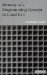# List of Figures## Chapter 2: From Source File to Executable File

Figure 2.2: Layout of a C/C++ source program and static objects
Figure 2.3: Object module structure
Figure 2.4: Object module (simplified) of the sample program
Figure 2.5: Creation of load module
Figure 2.7: From source program to its "placement" in memory during execution
Figure 2.8: Dynamic memory allocation

## Chapter 3: Variables and Objects; Pointers and Addresses

Figure 3.1: Overflow
Figure 3.2: A structure improperly placed in memory
Figure 3.3: A structure properly placed in memory with the use of padding
Figure 3.4: A pointer points to a memory location
Figure 3.5: A pointer points to a "virtual data container"
Figure 3.6: What is the value stored in the four bytes starting at address 802340?
Figure 3.7: Looking through char* " glasses " at a char virtual data container
Figure 3.8: Looking through short* "glasses" at a short virtual data container
Figure 3.9: Looking through int* "glasses" at an int virtual data container
Figure 3.10: Looking through float* "glasses" at a float virtual data container

## Chapter 4: Dynamic Allocation and Deallocation of Memory

Figure 4.1: Two- tier memory management
Figure 4.2: Fragment of a binary tree
Figure 4.3: Node c was extended but has not moved
Figure 4.4: Node c was extended and has moved

## Chapter 5: Functions and Function Calls

Figure 5.1: Flow of control during function calls
Figure 5.2: A general activation frame

## Chapter 6: One-Dimensional Arrays and Strings

Figure 6.1: Concept of one-dimensional array
Figure 6.2: Accessing a one-dimensional array via a pointer
Figure 6.3: Representation of the int x array
Figure 6.4: The array x is accessible from the function doit()
Figure 6.5: Dynamic array (Exercise 6.8)

## Chapter 7: Multi-Dimensional Arrays

Figure 7.1: Visualization of the two-dimensional array x
Figure 7.2: The row-major storing format of a two-dimensional array x
Figure 7.3: The "slice" when the first index is fixed as 1
Figure 7.4: The "slice" when the second index is fixed as 2
Figure 7.5: The "slice" when the third index is fixed as 2
Figure 7.6: Dynamically created two-dimensional array p

## Chapter 8: Classes and Objects

Figure 8.1: An object and its encapsulation
Figure 8.2: Memory allocation for sample
Figure 8.3: Memory allocation for global sample and sample1
Figure 8.4: Memory allocation for local sample

## Chapter 9: Linked Data Structures

Figure 9.1: A node with 'a' stored in it
Figure 9.2: A compacted node with 'a' stored in it
Figure 9.3: Compacted tree from the example
Figure 9.4: Compacted tree from the example after relativization

## Chapter 10: Memory Leaks and Their Debugging

Figure 10.1: Modifying a C program in order to trace memory leaks
Figure 10.2: Modifying a C program that has an external object code in order to trace memory leaks

## Chapter 11: Programs in Execution: Processes and Threads

Figure 11.1: Command-line argument structure for prog Arg1 Ar2
Figure 11.2: Command-line argument structure for prog A B3 C45
Figure 11.3: A single thread of execution
Figure 11.4: Two different threads of execution

## Appendix One: Hanoi Towers Puzzle

Figure A.1: Hanoi towers puzzleMemory as a Programming Concept in C and C++
ISBN: 0521520436
EAN: 2147483647
Year: 2003
Pages: 64

Similar book on Amazon